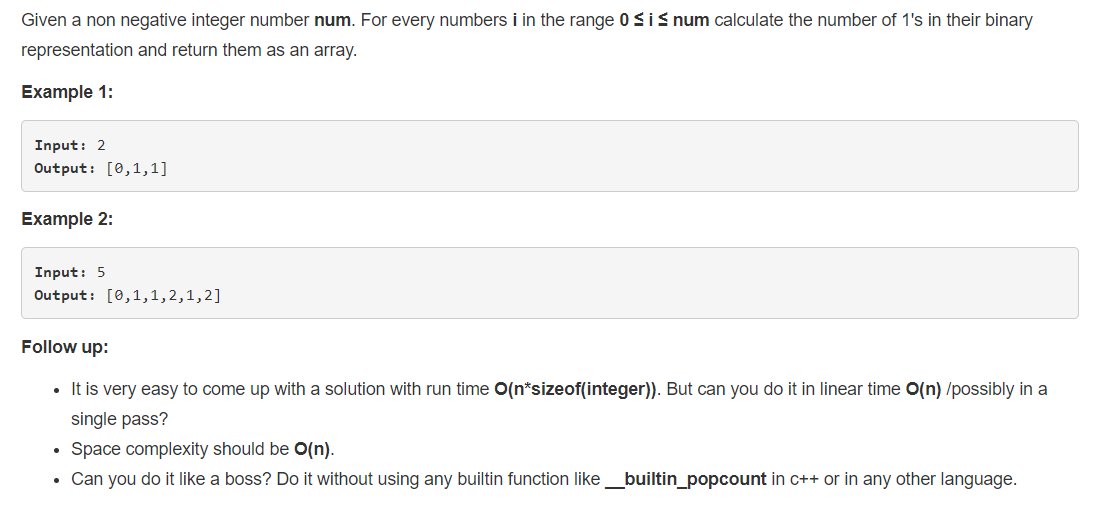# 338. Counting Bits

1、审题2、分析

给出数字 num， 求出 0~num 的每个数字的二进制形式中包含的 字符 ‘1‘ 的个数。

1、思路

方法一、

依次计算 1~n 每个数字的二进制形式中包含的 ‘1‘ 的个数。

    public int[] countBits(int num) {
int[] result = new int[num + 1];
for (int i = 1; i <= num; i++) {
int tmp = i, count = 0;
while(tmp > 0) {
count++;

if(tmp == 1)
break;
tmp &= (tmp - 1);
}
result[i] = count;
}

return result;
}


方法二、

采用 f[n] = f[n >> 1] + (n & 1) 计算数字 n 包含的 ‘1’ 的个数。

其中 f[n >> 1] 记录的是 n 的二进制形式的前一部分（除了最后一个字符）

n & 1 : 记录的是最后一个字符是否为 1

    public int[] countBits(int num) {
int[] f = new int[num + 1];
for (int i = 1; i <= num; i++)
f[i] = f[i >> 1] + (i & 1);
return f;
}


posted @ 2019-07-01 11:12  skillking2  阅读(34)  评论(0编辑  收藏